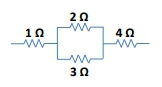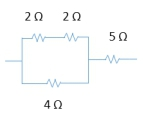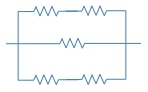## Physics

Learn the toughest concepts covered in Physics with step-by-step video tutorials and practice problems by world-class tutors

27. Resistors & DC Circuits

# Combining Resistors in Series & Parallel

1
concept

## Combining Resistors in Series & Parallel12m
Play a video:
2
Problem

What is the equivalent resistance of the following combination of resistors?3
concept

## Shortcut Equations for Resistors Parallel7m
Play a video:
Hey, guys. So in this video, I'm gonna show you some awesome shortcuts that you can use in some cases when you have resistors in parallel and this is gonna keep you from having to do a lot of work with fractions, let's check it out. All right, So if you have two resistors in parallel, there's a shortcut equation you can use. Now, remember, the general equation is that the equivalent resistance in parallel is one over our equivalents equals to one over R one plus one over R To this is if you have two resistors, this is the general equation, okay? And I'm gonna solve for our equivalents so that you never have to do this again. And you could just use a shortcut if you have two resistors, which is the most common thing you're gonna get. So the first thing if you remember we have to do is get a common denominator. And to do that, you're just gonna multiply our to here and then multiply our one here. So are one times are too. So if I do in the bottom, I also have to do in the top or two, and then are two times are one times are one. Let's extend the little fraction thing. So what happens now is I have are one or two in the bottom are one or two in the bottom. So I have a common denominator. So I can write our one times are too. And then at the top I have are two plus are one or I'm gonna ride R one plus R two. So it's in order. Are we done Know? Remember, we have to flip the sides. So if I flip here, I get our equivalents over one or simply are equivalent equals. And then if I flip the left, I gotta flip the right and this is gonna look, I'm sorry. This was a two right there. Hopefully caught that are one times are too over our one plus our two now notice. I'm drawing the r one and r two in the bottom really far apart. And the reason I'm doing this is because one of the biggest problems with this equation is that people forget whether the times is on top or the pluses on top. You dont know what goes where you might forget the way I remember which is silly but works is because this is a dots. It's a tiny dots. The R one r two are really close together, and this is a fat plus sign, which takes up a lot more space. The bottom variables are farther apart, and the skinny top and fat bottom gives you sort of a triangle. Okay, super silly. But maybe hopefully it works for you. Whatever works, right? So that's the equation. So whenever you have two resistors in parallel, you can just use that equation instead of playing with fractions. Okay, Now, super important is that you cannot do this for three or more resistors, so I actually want you to draw right This here. This is wrong, but I want you to write it. Let's say you might want to think that you can multiply are one or two. Why not just add an R three here and then at the bottom here, do a plus R three. Well, this is wrong. This does not work. So I want you to write it, scratch it out and say, Don't okay, do not do this. This Onley works for two resistors. It does not work for more than two. Okay, eso let me give you a super quick example here. Let's say you have a four and a six, and you want to combine them into a single resistor. You would just use this equation here and say that the equivalent resistance is four times six, divided by four plus six. I'm setting up the apprentices so I can put the numbers inside +4646 And this is 24 divided by 2.4, right, much faster than play with fractions. So that's the first shortcut. Um, the most important thing always is that you know the general equation, because this is gonna work for everything. But the shortcut is pretty handy as well. It's more important to shortcut number two, but shortcut number two is super simple. If you have resistors of the same resistance, you can also use a shortcut equation. So let's say I have a Let's make it creepy. 66 and six. Let's say you have something like this. What's the equivalent resistance? Well, if you write the the general equation, remember, you cannot write this equation right here. The one we just talked about because that works only for two. But if you have this, you end up with something like this 1/6, plus 1/6, plus 1/6. And the denominators already the same to end up with 3/6 or 6/3, which is to the fast way to have done this is to just say that the equivalent resistance when they're all the same in parallel is just the same resistance which in this case, is six divided by the number of resistors. So you could have just done six divided by three is too. For example, if you have eight, eight, eight, and eight the equivalent resistance here his super easy to calculate its AIDS and there are four of them. The equivalent resistance is just a to cool. So now I'm gonna do an example. That sort of merges all these ideas. You not know how to easily combine things that are the same. And you know how to easily combine when they're the same resistance and you know how to easily combine. If there's two of them using that first equation now, you can actually use those two rules to your advantage. So if you get a question like this. This might look Harry, but it's actually really simple. So we're going to do notice we have a nine a nine in the nine. And even though they're not right next to each other, you could technically rearrange them to be right next to each other. And you can say, You know what? This nine with this nine and this nine because they're the same, I can write. The equivalent resistance of the three nines is just 9/3. I'm using this shortcut right here. There are three nines, so it's just nine divided by three, which is three. Okay, you can do the same thing for the 12. There's 2 12 so I can say the equivalent resistance of the combined are gonna be 12 divided by two just six. So what I can do is all the Reds become a simple a single three and the and the blue becomes a six. And now, if I combine these two because I have two resistors that are parallel to each other, right, let's put the little connectors this way. So there's two resistors here. There are parallel to each other. I can use the first shortcut equation, which is You multiply at the top and then you add at the bottom. Remember the pyramid, right? It's multiply, um, and add. So this is gonna be three times 63 plus times six, of course, is 18 3 divided three plus six is nine. Silly answer here is to homes. So notice how we're able to combine everything really, really quickly. 1993 12 12 is a six. Put them together and you get a two. All right, that's it for this one. Let's get going.
4
Problem

What is the equivalent resistance of the following combination of resistors?5
example

## Weird Arrangement (Re-Drawing Resistors)6m
Play a video: